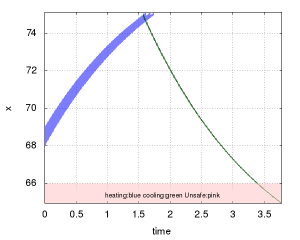# Linear Thermostat

Linear Thermostat  is a simple hybrid system simulating the temperature control system of the building.

## Dynamics

### MODE 0

$$\left \{ \begin{array}{l} \dot x = 40-0.5 x \end{array} \right.$$

invariant

$$x \leq 75$$

### MODE 1

$$\left \{ \begin{array}{l} \dot x = 30-0.5 x \end{array} \right.$$

invariant

$$x \geq 65$$

## Properties

Please see details in the attached file

##Link to the example file: linThermo.hyxml

references:

 Fehnker, Ansgar, and Franjo Ivančić. “Benchmarks for hybrid systems verification.” In Hybrid Systems: Computation and Control, pp. 326-341. Springer Berlin Heidelberg, 2004.